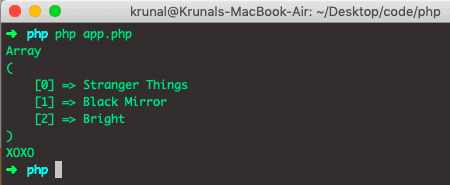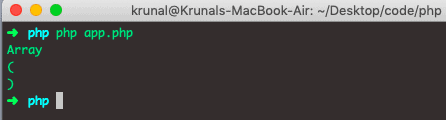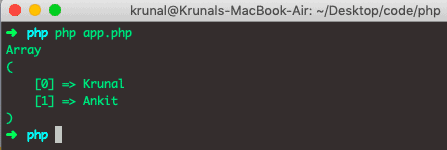# PHP Array Pop: How to remove array elements in PHP

If you do not know how to add the values to an array, check out PHP array_push(). NULL will be returned if an array is empty (or is not an array). An array_pop() function will produce an error of level E_WARNING when called on a non-array.

## PHP Array Pop

PHP array_pop() is a built-in function that removes the last element of an array. The array_pop() function deletes the last element of an array. The array_pop() function Pops the element off the end of an array.

### Syntax

See the following syntax of array_pop() function.

`array_pop(array)`

### Arguments

An array argument is required, which is the array whose elements will be removed.

### Example

See the following example.

```<?php

// app.php

\$netflix = ['Stranger Things', 'Black Mirror', 'Bright', 'XOXO'];
\$removedElement = array_pop(\$netflix);

print_r(\$netflix);
echo \$removedElement."\n";
```

The output of the code is following.The array_pop() function always returns the removedElement.

It reduces the size of an array by one since the last item is removed from an array.

Let’s see the example where an array is empty.

```<?php

// app.php

\$netflix = [];
\$removedElement = array_pop(\$netflix);

print_r(\$netflix);
echo \$removedElement;
```

The output is following.## PHP Array unset()

To remove an individual element from an array, use the unset() function. The unset() function does not return anything; it removes the element from the specified index from an array.

See the following example.

```<?php

// app.php

\$netflix = ['Krunal', 'Ankit', 'Rushabh'];
unset(\$netflix);

print_r(\$netflix);
```

The output is following.So, it has removed the 2nd indexed element, which is Rushabh.

So, if we want to remove the last element, we can use the array_pop() function, and if we’re going to remove an element at the specified position, we can use the PHP array unset() function.

Notice that the unset() function leaves a gap in our numerically indexed array. That means if we have an array of length 6 and remove an index of 4, then the remaining array has 0, 1, 2, 3, 5, 6. This is because the 4th index will be removed from an array.

That’s it for this example.

Categories PHP

This site uses Akismet to reduce spam. Learn how your comment data is processed.